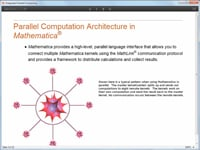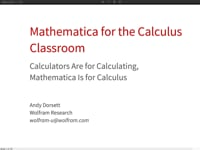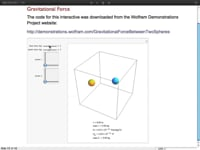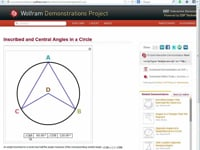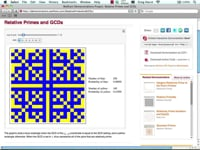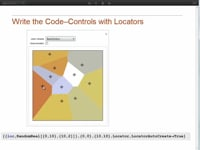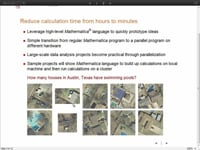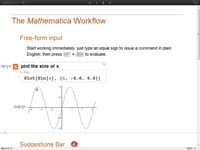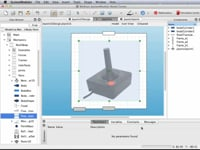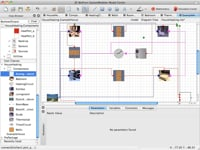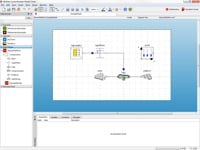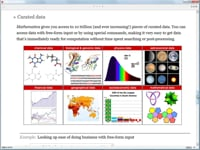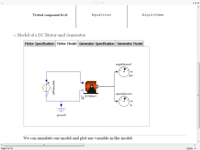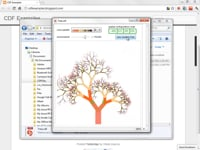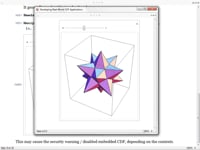Learn how »

# Wolfram SystemModeler and Mathematica: Quick Start

This course from the Wolfram SystemModeler Virtual Conference 2012 focuses on analyzing model equations and simulation results with Mathematica. You'll also learn about the link between Mathematica and SystemModeler.